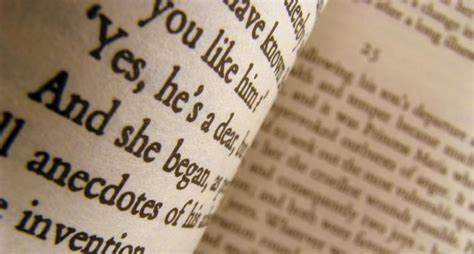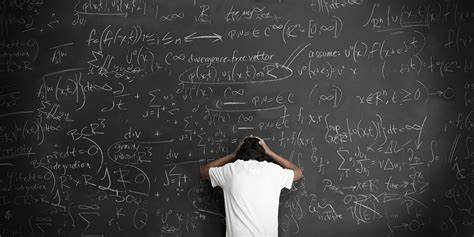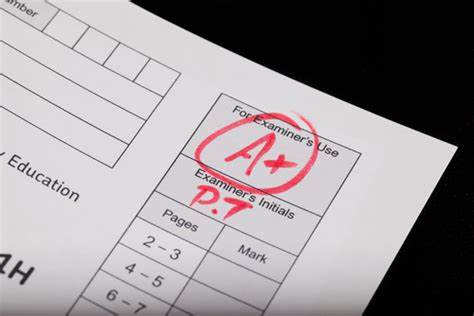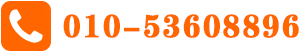# Motion I – Uniform Acceleration

Course1

This Factsheet will cover:

1the basic definitions of speed, velocity and acceleration

2 the use of the equations of motion for uniform acceleration

3 the application of the equations of motion to projectile motion

1.      Basic Concepts

Speed and velocity. Speed is the rate of change of distance.

The speed of a body at any instant is equal to the magnitude of its velocity at that instant. However, this is not generally the case for average speed and average velocity. To see why this is, imagine walking 5 m North in 2 s, then 5 m South in 2 s. Since you end up at the point where you started, your overall

displacement is zero – so your average velocity is zero. However, you have travelled a distance of 10 m in 4 s, so your average speed is: 10 /4 = 2.5 ms-1.

The average speed will only be the magnitude of the average velocity if the body concerned is moving in a straight line, without reversing its direction – since then, the distance it moves will always be equal to the magnitude of its displacement.

2.      Equations of motion for uniform acceleration

v = u + at            u = initial velocity

v2 = u2 + 2as          v = final velocity

s = ut + ½at2          a = acceleration

s = ½ (u + v)t         s = displacement from the starting point

t = time

3.  Approach to problems using equations of motion

1. Check that the body is moving with constant acceleration!

2. Write down any of u, v, a, s, t that you know

3. Note down which of u, v, a, s and t that you want (e.g. write a = ?)

4. Decide which equation to use by looking at which of the variables you have got written down in steps 2 and 3. For example, if you have got values for u, t and s, and you want a value for v, then you look for the equation with u, t, s and v in it.

5. Substitute the values you know in, then rearrange.

6. Check that the answer makes sense.

Course2

Typical example of Motion

Example 1.

A particle is moving in a straight line with constant acceleration. It passes point P with speed 2 ms-1. Ten seconds later, it passes point Q. The distance between P and Q is 40 metres. Find the speed of the particle as it passes point Q.

We know: u = 2ms-1 s = 40 m t = 10 s

We want: v = ?

Since we have u, s, t and v involved, use s = ½ (u + v)t

Substituting in:

40 = ½ (2 + v)10

40 = 5(2 + v)

40 = 10 + 5v

30 = 5v

v = 6 ms-1

Example 2.

A particle is moving in a straight line with constant acceleration 0.2 ms-2. After it has moved a total of 20m, its speed is 8ms-1. Find its initial speed

a = 0.2 ms-2 s = 20m v = 8 ms-1 u = ?

So use v2 = u2 + 2as

82 = u2 + 2(0.2)(20)

64 = u2 + 8

56 = u2

u = 7.48 ms-1 (3SF)

Tips:

1. It is usually easier to put the values in before rearranging the equations.

2. Take particular care with negative values. On many calculators, if you type in –22, you will get the answer –4, rather than the correct value of 4. This is because the calculator squares before “noticing” the minus sign.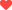喜欢 

## 最新文章 More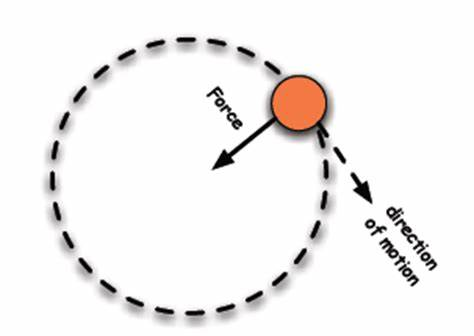## 相关推荐#### A-level基础数学知识串讲

A-level基础数学知识串讲.....

## 猜你喜欢 More